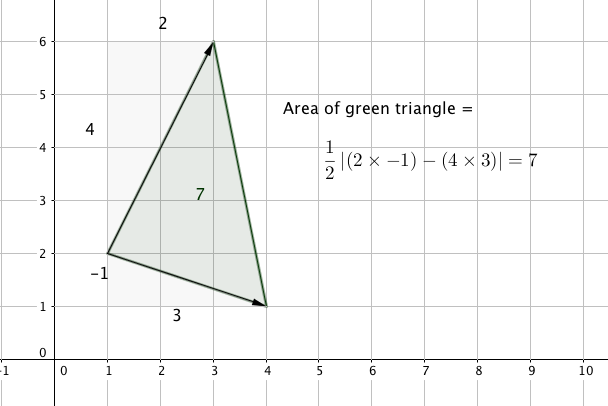# Vectors in 3D: Triangle Project

I use this lesson as a hook into vector algebra and as an introduction to working in 3D space. The idea is, they have to do quite a bit of work to find the area of their triangles. To conclude, we do some ‘number magic’ (the as yet unstudied vector algebra) to calculate the area, which illustrates the efficiency of vector algebra and hopefully garners interest and motivation to figure out why it works.

# Preliminary knowledge:

1. Distance formula/Pythagoras Theorem when working in 3D, or ability to figure it out during the task;
2. Cosine rule to find an angle;
3. Area formula for a triangle,# Resources:

1. A room with little or no furniture;
2. Chalk or masking tape for marking axes on the walls;
3. Rope – Several 2 – 3 meter lengths. I suppose thick, colourful wool would work.
4. Geogebra open on a laptop, or the applet below, one per group of three students.

# Preparation:

Move furniture to the side of the room, choose one corner of the room to use asaxes. Mark from 0 to 10 on each axes with chalk, or write on tape. Remember to label each axes.

Group students into groups of 3. Can work with some creativity with 2.

# Activity:

Each student chooses a point in the room, and takes a note of the coordinates. In their groups of 3, students ‘make’ the triangle, using wool/string/rope.

Students determine (estimate) by sight, which triangle is largest, which has the smallest angle.  They can confirm their estimates later. Is the triangle a 2D shape or a 3D shape? If a ball were to be rolled on their triangle from the highest vertex, in which direction would it roll? Did any group choose three points that are colinear? Can they figure out three points that are colinear?

Now that they have seen their triangles, in groups of three they are to calculate the area of the triangles using previous knowledge – distance formula, cosine rule for angle, area of triangle. This part of the project takes a good amount of time.

When they have written up the area of their triangle, say, on the whiteboard, they can then go and draw their triangle on geogebra.  Input points using the inpute bar, with command such as A=(3,4,5). They should troubleshoot their calculations if their area doesn’t agree with the area given by geogebra.

Here is a short video on how to use the applet:

The applet:

Finally, the teacher can demonstrate finding the area of the triangle using vector algebra.

# Homework

Using the 2D graphics on geogebra, create another demonstration of the following calculation:Use the ‘object properties’ ‘label’ ‘value’ to verify your calculations are correct.

# Following up

In a following lesson, we generally follow up on this lesson with some formal mathematics. We begin with componentsfor vectors,, andrespectively, and follow through the formula Area, until all components have simplified toAs a homework to this lesson, students are invited to write their own proof for the triangles drawn on theplane as shown in the ‘homework’ task above.